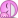# What are the frequencies of the fundamental and first two overtones?

jlwood13

## Homework Statement

A guitar string is 90.0 cm long and has a mass of 3.17 g. From the bridge to the support post is 60.0 cm and the string is under a tension of 506 N. What are the frequencies of the fundamental and first two overtones? (f1,f2,f3)

## Homework Equations

v=sqrt(FT/m/l)
v=$$\lambda*f$$

## The Attempt at a Solution

I checked in my text and found that for f1, l=1/2$$\lambda$$1; for f2, l=$$\lambda$$2; and for f3, l=3/2$$\lambda$$3. I tried using 60.0 cm (and converted to meters) and 90.0 cm (converted to meters) and 30.0 cm (converted to meters) for l, but nothing worked. I also converted 3.17 g into kilograms so that's not an issue. I'm not sure what to do now..

Ofey
The fundamental tone is heard when the length of the guitar sting equals half the wavelenght (corresponding to the fundamental tone).

Due to the construction of the guitar, you will get a node in both ends, and the distance between 2 adjacent nodes is lamda/2.

EDIT:

My error, I didn't read the assignment carefully. You have to use 60 cm and not 90 cm.

Last edited:
Chewy0087
Yeah for the fundamental 60cm is only 1/2 of it. - Wavelegnth for the fundamental = 120cm.

I'm struggling to see what you've done wrong, I think it might just be a case on number crunching wrong. Bear in mind the wavelegnth figure you'd use is 2x 60cm, however the mass per unit legnth you'd use is 90cm... What do you mean "nothing worked" what sort of answers were you getting? Bear in mind that if it's 506N the speed would have to be damn fast :P, I get a very high speed

edit; welcome to pforums ;p

Ofey
I am not totally sure on this, but since it states that the whole string has a mass of 3.17 gram, doesn't that mean that the 60 cm has a smaller mass? If the answer is wrong it might be because of the wrong value on mass.

EDIT:

I didn't notice that this was exactly what Chewy said, I am sorry.

Last edited:
Chewy0087
That makes no difference, you're trying to work out the mass per unit legnth, since the whole string weighs 3.17 grams, & the whole string is 60cm long your calculation must be

0.00317 / 0.9 to work out m0, but again, what sort of answers are you churning out?

Last edited:
Ofey
Isn't it 0.00317/0.9 since it says that the whole string has the mass 3.17 grams?

Chewy0087
Yah, that's what i meant sorryjlwood13
When dividing my the unit mass I used .6 instead of .9. It was an error in number crunching. Thanks for your help! Sorry that I didn't respond sooner. Also, thanks for the welcome!